Tuesday, March 1, 2011

555-logic: designing digital circuits with 555s only!

The basic idea of 555-logic is to implement digital logic circuits with 555 ICs only. This gives advantage that a relatively higher and wide range of power supply voltage can be used, limited by the specification of the 555 IC (4.5V - 16V). According to Shannon through his famous 1937 Masters thesis at MIT, any combinational logic circuits can be made with switches. With semiconductor technology, these switches can be in the form of transistors. To use 555 as a transistor switch, one can utilize the internal pull-down transistor of a 555 (Figure 1).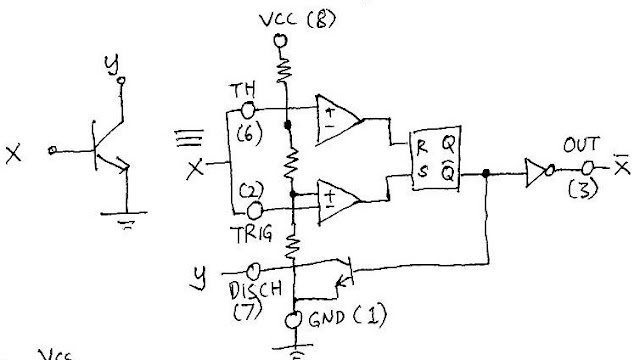Figure 1  Using the transistor of a 555

There is a restriction on how to use this transistor as its emitter is connected to ground pin (4). Even though one may be tempted to connect that emitter (or ground pin) to something other than ground voltage, this will not guarantee to work since the ground voltage is needed by other parts of the 555 IC to function. Therefore, the use of the 555 transistor as a switch is restricted to the implementation of pull-down network of switches with no cascading of emitters to collector of another transistor. This leaves a reasonable choice to implement a NOR gate. A NOR gate can be made with two pull-down transistors with collector pins connected together forming the output. A pull up resistor is to be connected between these collector pins and VCC, and a 555 can be used for this since a series of three 5ks resistors exist in a 555. The implementation of such a NOR gate with 555 is shown in Figure 2.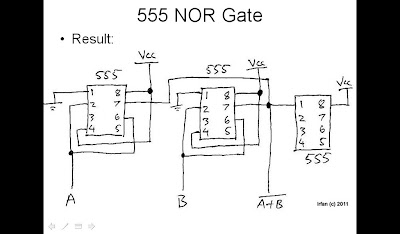Figure 2  Implementation of NOR gate with 555
A NOR gate is said to be a minimum set for implementing logic functions, since NOR gates can be made into any other logic gates. But it still would be better if other form of basic logic gates made from pure 555 can also made. One obvious logic gate with a 555 is the inverter, which has actually been shown above in Figure 1 (see pin X').
To implement digital sequential systems or state machines, a form of memory such as a clocked flip-flop is needed. A 555 chip contains an RS flip-flop, so it makes real sense to try to make use of it as a clocked flip-flop. The main difficulty on using this internal flip-flop is that the R and S inputs are fixed to other components inside the 555, namely the comparator outputs. By carefully controlling the signaling of input pins connected to those comparators, one can obtain a level-triggered as well as edge-triggered D flip-flop with a 555. A positive level enabled D flip-flop with 555 is shown in Figure 3. To make this edge triggered, we can actually make a master-slave arrangement but since this would require a larger amount of 555s, in this work we built a short pulse generator instead to make the level-triggered flip-flop into an edge-triggered one. The circuit on Figure 4 is a negative-edge triggered D flip-flop designed using the above approach.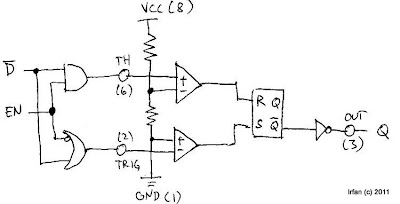Figure 3  Level-enabled D-flip flop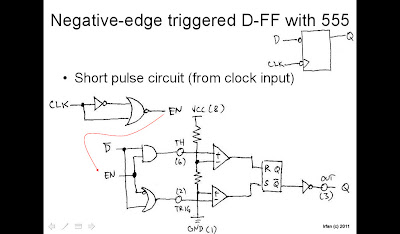Figure 4  Negative edge triggered D flip-flop
The logic between data and clock sources inputs with the 555 can be made with 555 logic (using NOR and NOT gates), and the resulting circuit realization is shown in Figure 5.Figure 5  A 555-logic-oriented implementation of circuitry for D flip-flop with 555.
With these NOR gate, NOT gate, and a edge triggered D flip-flop made solely from 555, it is time now to test this concept in real scenario. An added bonus for this 555-logic concept is that the same 555 can be used as the clock source.

Case study: synchronous 2-bit grey code counter
Let us now consider a 2-bit grey-code counter that counts in this following order: 00, 01, 11, 10. A synchronous topology is chosen in this case study to emphasize the synchronously clocked sequential digital circuitry can be made. Using a general state machine topology, where states are fed back to flip-flops that holds the state information, through some combinational logic block, we can try to implement the following truth table:
Q1  Q0    NextQ1  NextQ0

0    0        0             1
0    1        1             1
1    0        0             1
1    1        1             0

Using sum-of-product and simplifications principles, we can get:
NextQ1 = Q0
NextQ0 = not Q1

The implementation of the above logic function can therefore be made as seen in Figure 6.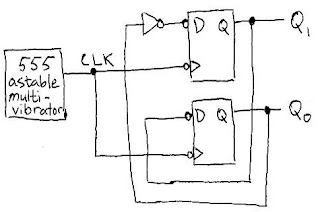Figure 6  Test circuit of synchronous 2-bit grey code counter

The circuit on figure 6 implemented with only 555s (except timing R and C for clock generation and series R for LED indication on outputs) is shown in Figure 7. The clock generator is a 555 astable multivibrator circuit with R1 = R2 = 1k2, and C = 100uF.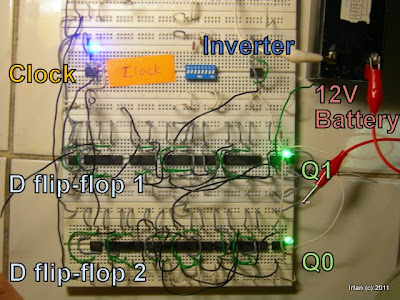Figure 6  A 2-bit synchronous grey-code counter implemented with 555s.

A video showing real experiment of the circuit can be seen in the following video link: http://www.youtube.com/watch?v=jpmp9uvjrwU

Conclusion:
555-logic proposed in this work relies on NOR gates (needs three 555s, OR one 556 and one 555), NOT gates (needs one 555), and negative-edge triggered D flip-flop (four 556s and six 555s).

1.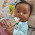judule hobi mikro kok isine basa inggris...hehe

2.@ayahnya dito: hehe iya sengaja dalam bahasa inggris mas, supaya bisa dipahami oleh lebih banyak orang (harapannya)... oh iya e blm diganti profile nya :D

3.maksude nek cocoke ketoke judulle kudune Hobby Micro dudu hobi mikro....hehehe

4.Ayo ditambahi tulisan neh, ben iso menang...

5.DEAR ALL:
More details about this 555 contest entry has been posted a week after this post. The original post has not been edited and left as it was since March 1st. See: http://hobimikro.blogspot.com/2011/03/more-about-555-logic-for-555-contest.html for the more complete explanation of the contest entry.

6.7.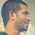What is diagram software you used to create these logic gate diagram. Is it creately ?